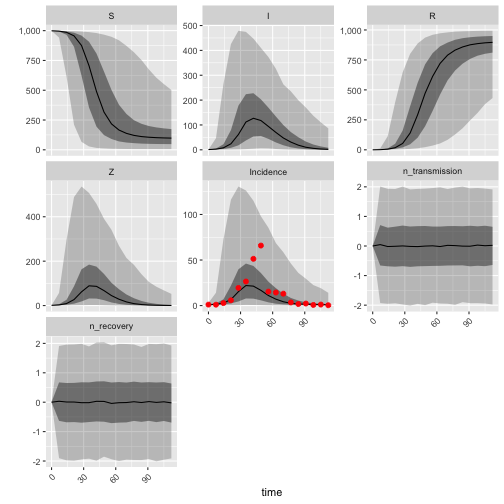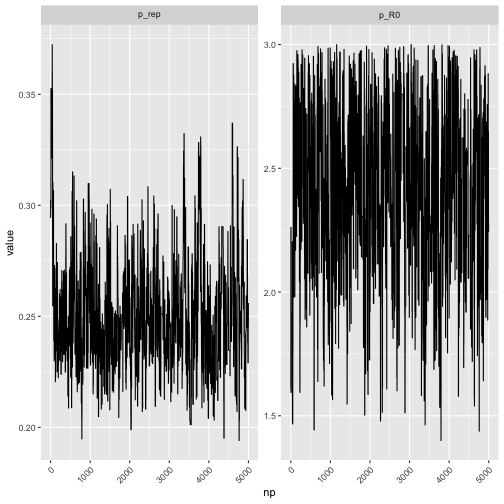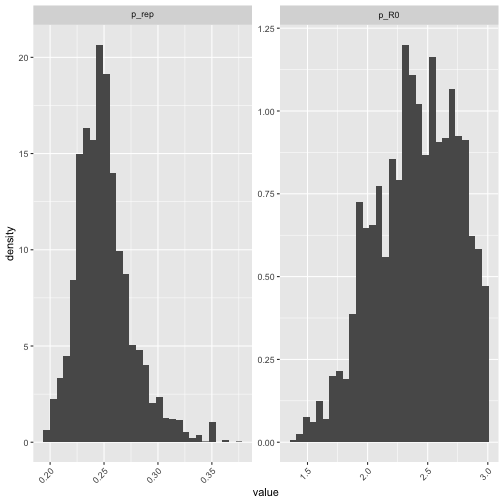# Introduction to RBi.helpers

Sebastian Funk on October 11, 2018

RBi.helpers is collection of helper functions to use with RBi, an R interface to LibBi, a library for Bayesian Inference.

This vignette builds on the RBi vignette, applying the higher-level functions contained in RBi.helpers to the same model introduced there. For the lower-level functions to run LibBi and read the results, please refer to the documentation and vignette that comes with RBi.

# Installation

The RBi.helpers package requires `R` (>= 3.2.0) as well as the packages:

• `rbi`
• `data.table`
• `reshape2`
• `lubridate`
• `coda`
• `ggplot2`
• `scales`
• `GGally`

Most functions also require a working installation of LibBi. Please see the RBi vignette for how to get one via homebrew or linuxbrew.

The package name of RBi.helpers is `rbi.helpers` (all lowercase). The current stable version can be installed using the `devtools` package:

``````# install.packages("devtools")
library('devtools')
install_github("sbfnk/rbi.helpers")
``````

Use

``````library('rbi')
library('rbi.helpers')
``````

These steps are reproduced from the RBi vignette, where there is more information on the individual steps

``````model_file <- system.file(package="rbi", "SIR.bi") # get full file name from package
SIRmodel <- bi_model(model_file) # load model
SIRdata <- bi_generate_dataset(SIRmodel, end_time=16*7, noutputs=16, seed=12345678)
``````

# Adapt the number of particles

In the RBi vignette, a stochastic SIR model is fitted to simulated data from the same model using particle Markov-chain Monte Carlo with 16 particles. Given a model and data, how do we know how many particles we need? This question does not have a simple answer, as the “optimal” number of particles may depend on the state of the Markov chain. A possible rule-of-thumb is to choose the number of particles such that the variance of the log-likelihood near the mode is approximately one. This suggests a strategy by which first and approximate location of the mode or mean of the posterior distribution is obtained in a trial run, before the numer of particles is adjusted by monitoring the variance of the log-likelihood while keeping the parameters fixed. RBi.helpers implements the second part of this strategy (adjusting the number of particles at a given location in parameter space) with the `adapt_particles` method. For the first part (finding the mode), a crude method is to take a fixed number of samples from the prior distribution and choose the one that maximises the posterior distribution. In RBi, this can be achieved with

``````bi_prior <- sample(proposal="prior", SIRmodel, nsamples=1000, end_time=16*7, nparticles=16, obs=SIRdata, seed=1234)
``````

By using `proposal="prior"` we set the prior distribution to be the proposal distribution. In other words, when sampling from the posterior the proposals will be drawn independently from the prior distribution. Note that we set a seed to make the results reproducible. It is worth trying the commands with a different seed and seeing the difference to the results obtained below. The location in parameters of the sampler at the end of the 1000 samples will give an approximation of the mode of the posterior distribution. This can then be used to adjust the number of particles using

``````adapted <- adapt_particles(bi_prior)
``````
``````## Thu Oct 11 00:45:53 2018 Adapting the number of particles
``````
``````## Thu Oct 11 00:47:19 2018 16 particles, loglikelihod variance: 1.75766739956752
``````
``````## Thu Oct 11 00:47:34 2018 32 particles, loglikelihod variance: 0.849351565223584
``````
``````## Thu Oct 11 00:47:34 2018 Choosing 32 particles.
``````

This will take the last sample of the output file contained in the `libbi` object `bi_prior`, and use it to adjust the number of particles by starting with 1 particle (or a given `min`) and doubling it until the variance of the loglikelihood crosses 1. The number of particles is then saved in the `adapted` object:

``````adapted\$options\$nparticles
``````
``````##  32
``````

Having adjusted the number of particles, the second important information to give the posterior sampler is the proposal distribution. This can, again, be obtained using a sequence of trial runs, whereby the proposal distribution is sequentially adjusted from previous samples to be proportional to the empirical covariance of the posterior samples. The way this is implemented in the `adapt_proposal` function in RBi.helpers is that the proposal distribution is adjusted to come from a multivariate normal taking into account the covariance of samples obtained so far, until the acceptance rate lies between the given minimum and maximumad. For example, to adjust the proposal distribution for an acceptance rate between 0.05 and 0.4, we can run:

``````adapted <- adapt_proposal(adapted, min=0.05, max=0.4)
``````
``````## Thu Oct 11 00:47:34 2018 Adapting the proposal distribution
``````
``````## Thu Oct 11 00:47:34 2018 Initial trial run
``````
``````## Thu Oct 11 00:48:58 2018 Acceptance rate 0.407407407407407, adapting size with scale 1
``````
``````## Thu Oct 11 00:50:08 2018 Acceptance rate: 0.376376376376376
``````

The adjusted proposals are stored in the `bi_model` contained in the `libbi` object `adapted_proposal`

``````get_block(adapted\$model, "proposal_parameter")
``````
``````##  "p_rep ~ truncated_gaussian(mean = p_rep, std = 0.02, lower = 0, upper = 1)"
##  "p_R0 ~ truncated_gaussian(mean = p_R0, std = 0.4, lower = 1, upper = 3)"
``````

Note that the multivariate normal is realised as a series of conditionally dependent draws from normal distributions. The model can be saved via

``````write_model(adapted\$model, "SIR.bi")
``````

The steps above are exactly the steps used to create the proposal blocks in the file `SIR.bi` contained in the RBi package and used in its vignette.

# Plot libbi objects

Let us run take 5,000 samples from the posterior distribution of the model using the adapted proposal and number of particles, and generate sampled observations:

``````posterior <- sample(adapted, nsamples=5000)
pred <- predict(posterior, noutputs=16, with=c("transform-obs-to-state"))
``````

Note that we called `predict` with the `with=c("transform-obs-to-state")` option to also generate posterior predictive samples from of the observations.

Instead of using the coda routines for plotting as described in the RBi vignette, one can use the `plot.libbi` function. If passed a `libbi` object, it can be called using `plot`. Here, we use it with the `pred` object. We could also pass the `posterior` object, but that would not have the observation samples.

``````p <- plot(pred, plot=FALSE)
``````
``````## Warning in plot.libbi(pred, plot = FALSE): Correlations plot currently
## deactivated because of problems with the 'GGally' package
``````
``````## Warning in plot.libbi(pred, plot = FALSE): Pairs plot currently deactivated
## because of problems with the 'GGally' package
``````

There are various options available for controlling the plots. See `?plot_libbi` for a description of all the different available arguments.

The returned object `p` contains a number of different plots:

``````names(p)
``````
``````##  "trajectories" "densities"    "traces"       "data"
``````

## Trajectories

``````p\$trajectories
``````This is the default plot displayed when `plot_libbi` is called without `plot=FALSE`. Note that this plots the data on top of the observation trajectory, because we called `predict` with `with=c("transform-obs-to-state")`.

## Trace plot

``````p\$traces
``````## Posterior densities

``````p\$densities
``````
``````## `stat_bin()` using `bins = 30`. Pick better value with `binwidth`.
``````This plots histograms. Smooth density interpolations can be plotted by passing `densities="density"` to `plot_libbi`.

## Summary data

All the data used for the plot are stored in the `data` object:

``````p\$data
``````
``````## \$observations
##     time      value       var time_next min.1 max.1 min.2 max.2  np
##  1:    0  1.0627469 Incidence         1     0     0     0     0 n/a
##  2:    7  0.9579713 Incidence         8     0     0     0     0 n/a
##  3:   14  2.7424396 Incidence        15     0     0     0     0 n/a
##  4:   21  5.8324430 Incidence        22     0     0     0     0 n/a
##  5:   28 19.4283761 Incidence        29     0     0     0     0 n/a
##  6:   35 26.4151382 Incidence        36     0     0     0     0 n/a
##  7:   42 51.3084677 Incidence        43     0     0     0     0 n/a
##  8:   49 65.8505966 Incidence        50     0     0     0     0 n/a
##  9:   56 15.3140031 Incidence        57     0     0     0     0 n/a
## 10:   63 14.3198948 Incidence        64     0     0     0     0 n/a
## 11:   70 13.0396847 Incidence        71     0     0     0     0 n/a
## 12:   77  3.4399803 Incidence        78     0     0     0     0 n/a
## 13:   84  1.7107317 Incidence        85     0     0     0     0 n/a
## 14:   91  2.2267790 Incidence        92     0     0     0     0 n/a
## 15:   98  0.7146317 Incidence        99     0     0     0     0 n/a
## 16:  105  1.0986011 Incidence       106     0     0     0     0 n/a
## 17:  112  0.3891336 Incidence       113     0     0     0     0 n/a
##
## \$trajectories
##             var time time_next         value       max.1       min.1
##   1:          S    0         1 999.000000000 999.0000000 999.0000000
##   2:          S    7         8 996.038554924 997.3764615 992.7667844
##   3:          S   14        15 986.035926961 993.0554255 965.1775254
##   4:          S   21        22 956.314112727 982.7408495 864.4673779
##   5:          S   28        29 875.380753127 958.6346203 622.2248272
##  ---
## 115: n_recovery   84        85  -0.010630534   0.6706826  -0.6685978
## 116: n_recovery   91        92   0.020885620   0.6817256  -0.6332691
## 117: n_recovery   98        99  -0.006243642   0.6702387  -0.6776544
## 118: n_recovery  105       106   0.013272406   0.6817972  -0.6388646
## 119: n_recovery  112       113  -0.021853816   0.6359736  -0.6939511
##           max.2      min.2
##   1: 999.000000 999.000000
##   2: 998.359411 941.976600
##   3: 997.234823 613.458310
##   4: 995.480661 210.331824
##   5: 992.505235  66.406758
##  ---
## 115:   1.879442  -1.967180
## 116:   1.983554  -1.933638
## 117:   1.972934  -2.010915
## 118:   1.995131  -1.955931
## 119:   1.929279  -1.911365
##
## \$params
##        distribution parameter   np     value varying
##     1:    posterior     p_rep    0 0.3024169    TRUE
##     2:    posterior     p_rep    1 0.3024169    TRUE
##     3:    posterior     p_rep    2 0.2944827    TRUE
##     4:    posterior     p_rep    3 0.2986428    TRUE
##     5:    posterior     p_rep    4 0.2986428    TRUE
##    ---
##  9996:    posterior      p_R0 4995 2.2792194    TRUE
##  9997:    posterior      p_R0 4996 2.2792194    TRUE
##  9998:    posterior      p_R0 4997 2.2792194    TRUE
##  9999:    posterior      p_R0 4998 2.2792194    TRUE
## 10000:    posterior      p_R0 4999 2.2432976    TRUE
``````

# Compute DIC

To compute the Deviance Information Criterion (DIC), use `DIC`:

``````DIC(posterior)
``````
``````##  86.10961
``````

# Create inference chains

In combination with the `magrittr` package, the it is possible to construct inference chains more elegantly. For example, to get posterior samples from adapted Metropolis-Hastings as above, we could simply write

``````library('magrittr')
posterior <- sample(proposal="prior", SIRmodel, nsamples=1000, end_time=16*7, nparticles=16, obs=SIRdata, seed=1234) %>%
sample(nsamples=5000)
pred <- posterior %>%
predict(noutputs=16, with=c("transform-obs-to-state"))
``````
``````## Thu Oct 11 00:53:05 2018 Adapting the number of particles
``````
``````## Thu Oct 11 00:54:26 2018 16 particles, loglikelihod variance: 1.05934226333476
``````
``````## Thu Oct 11 00:54:39 2018 32 particles, loglikelihod variance: 0.931450771667354
``````
``````## Thu Oct 11 00:54:39 2018 Choosing 32 particles.
``````
``````## Thu Oct 11 00:54:39 2018 Adapting the proposal distribution
``````
``````## Thu Oct 11 00:54:39 2018 Initial trial run
``````
``````## Thu Oct 11 00:55:49 2018 Acceptance rate: 0.377377377377377
``````